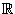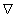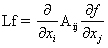18.152 | Fall 2005 | Undergraduate

# Introduction to Partial Differential Equations

## Assignments

### Partial Differential Equation Assignment 1

Assignment 1 as a (PDF)

#### Problem 1

Prove that a Harmonic function with an interior maximum is constant.

#### Problem 2

Write out the laplacian in planepolar coordinates.

#### Problem 3

A Green’s function onn is a harmonic function onn \{0} which depends only on the radius (for example log r on2). Find nontrivial Green’s functions for all dimensions.

#### Problem 4

The heat equation for a function u:× [0, ∞) is. Find all solutions of the form u = ƒ(t)g(x).

#### Problem 5

Find all solutions u of the heat equation on [0, 1] × [0, ∞) with the u = 0 on ({0} ∪ {1}) × [0, ∞).

### Partial Differential Equation Assignment 2

Assignment 2 as a (PDF)

#### Problem 1

Let u be a function on the ball B1(0) ⊂2 with ∫B1(0) |u|p <]infty for some constant p > 2. Show that u is holder continuous. [Hint: Use Morrey on ∫ 1.|u|2 ]

#### Problem 2

Let u:n, and define OSCBr(x)u = supBr(x)u - infBr(x)u. Show that if there is some constant 0 < γ < 1 with

oscBr(x)u ≤ γ oscB2r(x)u

for all x and all r then u is Holder continuous.

#### Problem 3

Let L be a uniformly elliptic 2nd order operator in divergence form takingLet u be a function with Lu ≥ 0, and Φ:a function with Φ’, Φ" ≥ 0. Show that L(Φ(u))≥ 0.

#### Problem 4

Let L be an operator as in question 3, and let u be an L harmonic function. Prove that |u|2 is holder contiuous. [This is likely to be difficult.]

Fall 2005
Problem Sets
Lecture Notes